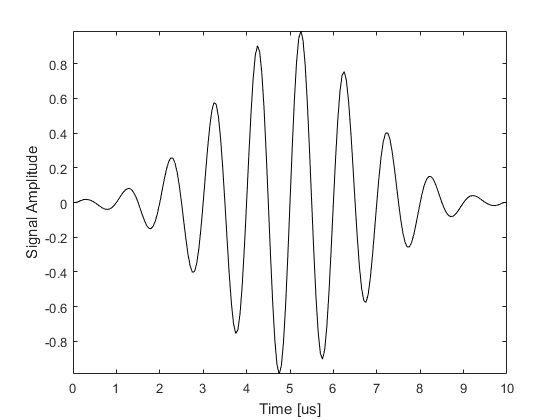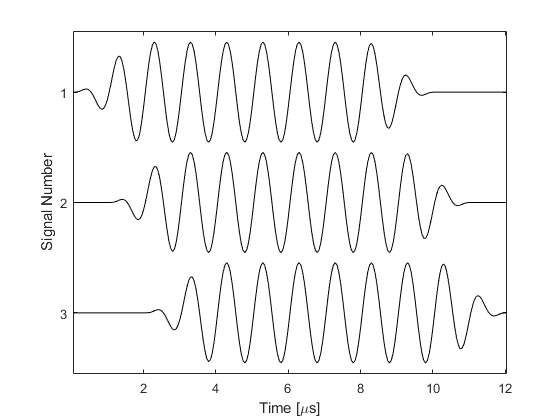# toneBurst

Create an enveloped single frequency tone burst.

## Syntax

```signal = toneBurst(sample_freq, signal_freq, num_cycles)
signal = toneBurst(sample_freq, signal_freq, num_cycles, ...)
```

## Description

`toneBurst` creates an enveloped single frequency tone burst for use in ultrasound simulations. If an array is given for the optional input `'SignalOffset'`, a matrix of tone bursts is created where each row corresponds to a tone burst for each value of the `'SignalOffset'`. If a value for the optional input `'SignalLength'` is given, the tone burst/s are zero padded to this length (in samples). Several examples are given below.

```% return and plot a 1 MHz tone burst with 10 cycles and a Gaussian envelope sampled at 20 MHz
toneBurst(20e6, 1e6, 10, 'Plot', true);
``````% specify several signals with different temporal offsets
sample_freq          = 20e6;
signal_freq          = 1e6;
num_cycles     	     = 10;
num_ring_up_cycles   = 2;
num_ring_down_cycles = 2;
signal_offsets       = [0, 20, 40];

% create signals
signals = toneBurst(sample_freq, signal_freq, num_cycles, 'Envelope', [num_ring_up_cycles, num_ring_down_cycles], 'SignalOffset', signal_offsets);

% plot signals
figure;
t_axis = (1:size(signals, 2)) / sample_freq;
stackedPlot(1e6 * t_axis, signals);
xlabel('Time [\mus]');
ylabel('Signal Number');
```## Inputs

 `sample_freq` sampling frequency [Hz] `signal_freq` signal frequency [Hz] `num_cycles` number of sinusoidal oscillations

## Optional Inputs

Optional 'string', value pairs that may be used to modify the default computational settings.

Input Valid Settings Default Description
`'Envelope'` `'Gaussian'`
`'Rectangular'`
`[num_ring_up_cycles, num_ring_down_cycles]`
`'Gaussian'` Envelope used to taper the tone burst. The last option generates a continuous wave signal with a cosine taper of the specified length at the beginning and end.
`'Plot'` (Boolean scalar) `false` Boolean controlling whether the created tone burst is plotted.
`'SignalLength'` (integer) `'auto'` Signal length in number of samples. If longer than the tone burst length, the signal is appended with zeros.
`'SignalOffset'` (integer) `0` Signal offset before the tone burst starts in number of samples.

## Outputs

 `signal` created tone burst

## Examples

`gaussian`